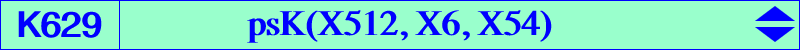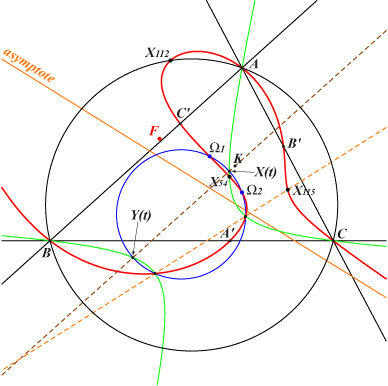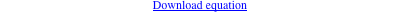too complicated to be written here. Click on the link to download a text file.X(54), X(112), X(115), X(826), X(9218), X(14885), X(32545) Brocard points A', B', C' : vertices of the cevian triangle of KConsider two points X(t), Y(t) with trilinear coordinates X(t) = cos(A + t) : : and Y(t) = sin(A + t) : : . These points lie on the Brocard axis. See Table 38. For any real number t, • X(t) and Y(t) are conjugated with respect to the Kiepert hyperbola, the locus of the isogonal conjugates of X(t) and Y(t). Note that the polar lines of X(t) and Y(t) in this hyperbola meet at X(5). the inscribed conic with perspector X(59), the isogonal conjugate of X(11). This conic is the locus of points with trilinear coordinates 1 ± cos(A ± t) : : or 1 ± sin(A ± t) : : . Note that the polar lines of X(t) and Y(t) in this conic meet at X(1). any conic of the pencil generated by these two latter conics. Their common points are X(39641), X(39642) and two other points on a line passing through X(2245) and two points whose barycentric product is X(1275) x X(1500), the barycentric square of X(4551) = X(37) x X(664), on the line X(6), X(2149). • the circle (C) with diameter X(t)Y(t) contains the Brocard points. This circle is orthogonal to the imaginary circle with diameter the imaginary foci X(39641), X(39642) of the Brocard inellipse. In other words, X(t) and Y(t) are inverse in this circle. Equivalently, Y(t) is the harmonic conjugate of X(t) with respect to X(39641), X(39642). Also, X(t) and Y(t) are Psi_X39 conjugates, see details here. Y(t) is also the reflection in X(39) of the inverse of X(t) in the circle with diameter X(3102), X(3103). Recall that X(3102), X(3103) and the Brocard points are the vertices of a square. • the circum-conic (C') passing through X(t), Y(t) contains X(54), the isogonal conjugate of X(5). • X(t), X(-t) and Y(t), Y(-t) are two pairs of points inverse in the Brocard circle. • X(t), Y(-t) and Y(t), X(-t) are two pairs of points inverse in the circle with diameter X(371), X(372). *** These two curves (C), (C') meet at two other points collinear with X(115) that lie on the cubic K629. K629 is a circular cubic with singular focus X(14675) whose isogonal transform is K630. These two cubics generate a pencil of circular circum-cubics passing through the Brocard points and two other (not always real) points which are the common points of the line X(110)X(512) and its isogonal transform. See a figure at the page K630. This pencil is invariant under isogonal conjugation and contains K021 = pK(X6, X512), nK(X111, X111, X523), nK(X187, X6, X110) and the isogonal focal cubic nK(X6, X187, X3906*) where X3906* is the isogonal conjugate of the infinite point X3906.